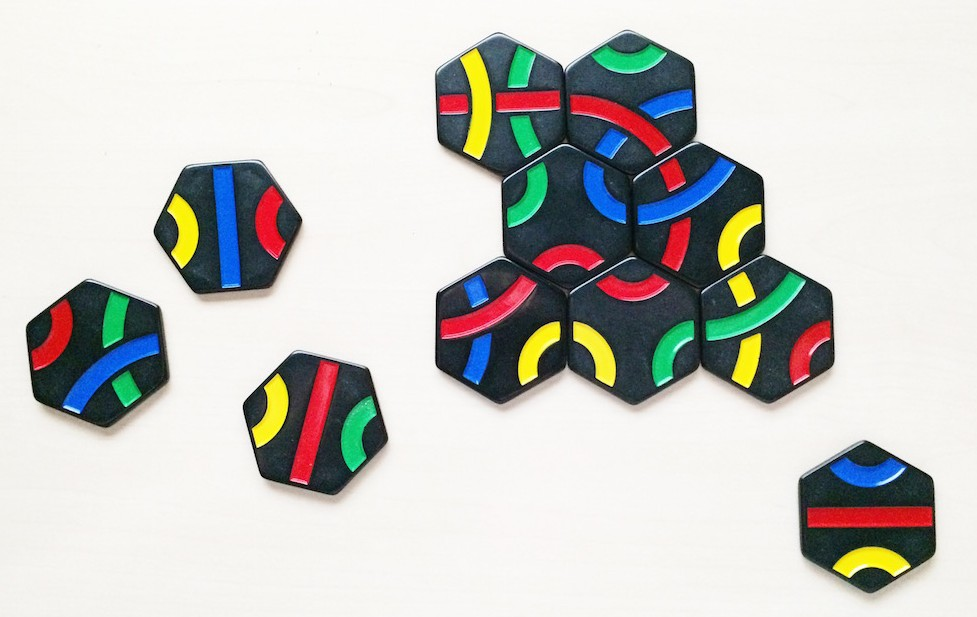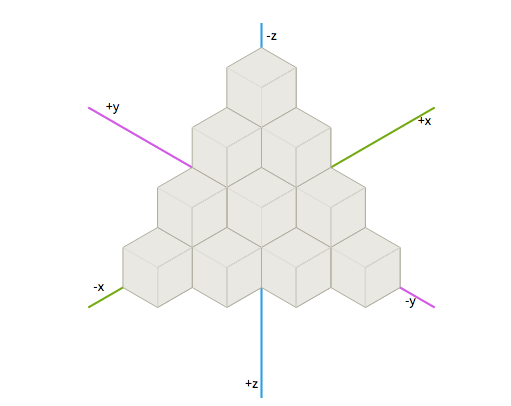# Jamie's Blog

Ruby developer. CTO. Swimmer. Always trying to write more

# Implementing HexGrids in RubyOne of my favourite board games is Tantrix. I can be played as a puzzle like Solitaire or as a competitive game. We picked it up in New Zealand over 10 years ago (it was invented there) and we played it in the evenings as we travelled around. The rules are pretty simple but the game can be frustrating and very tactical.

Anyway, I reckon that Tantrix (or Minesweeper, for that matter) would make a great compsci project instead of the usual projects: there’s a data structure representation problem, gameplay rules to be enforced and non-obvious rules of thumb to apply.

## Implementing Hex Space

The first step in a creating a game is representing the game state. For Tantrix we need to represent an arbitrary sized collection of hexagonal hexs. We can’t work off a traditional 2D square grid, instead we need a hexagonal grid. That’s when I discovered the amazing collection of work by Red Blob Games on HexGrids. The credit for all the maths and C++ implementations below goes to them; I wasn’t figuring it out from first principles.

Coordinates in hex-space are much like those in 3D space — because they are 3D coordinates. I’m using the ‘Cube Coordinate’ system which uses a constrained 3D space to represent hexagons. Seriously: go and check out the brilliant animation here.

http://www.redblobgames.com/grids/hexagons/#coordinatesOnce you get your head around the basic coordinate concepts, the implementation guide provides some C code illustrating the basic concepts.

### Making the Point

They use a `Hex` struct to represent coordinates in hex-space and perform operations on it. I decided that it made more sense to separate the location of something and the actual thing itself. So I have a `Point` class which is responsible for the location in hex-space.

Here’s the original C++ implementation

``````struct Hex { // Axial storage, cube constructor
const int q_, r_;
Hex(int q, int r, int s): q_(q), r_(r) {
assert (q + r + s == 0);
}

inline int q() { return q_; }
inline int r() { return r_; }
inline int s() { return - q_ - r_; }
};
``````

and my Ruby version

``````class Point

def initialize q, r, s = -q-r
@q = q
@r = r
@s = s
raise InvalidPointError unless valid?
end
``````

`q`, `r`, and `s` are our Hex coordinates (like, x,y,z in 3D space). The difference is that the `s` coordinate is technically unnecessary since it can be calculate from `q` and `r`. In Ruby we can easily provide an optional `s` argument which is calculated from the other constructor args.

It seemed easier for the moment to store `s` instead of calculating each time it’s required. I might still change my mind (and its easy to define a `s` method as `-q-r` but I generally prefer to store the variable as it makes debugging a little simpler.

### Equality

Next we need to define equality for `Point`. The C++ implementation looks like

``````bool operator == (Hex a, Hex b) {
return a.q == b.q && a.r == b.r && a.s == b.s;
}

bool operator != (Hex a, Hex b) {
return !(a == b);
}
``````

and in Ruby we can do

``````def == other
@q == other.q && @r == other.r && @s == other.s
end
alias :eql? :==

def hash
[@q, @r, @s].hash
end
``````

We implement equality as simply comparing the 3 coordinate values. We alias `eql?` to `==` so that we can use `Point` as keys in a `Hash`. Unlike C++, we don’t need to define `!=` because that shit is crazy.

Since we want to use `Point` in a `Hash`, we also implement the `hash` method which use the fairly common idiom of combining the values into an array and using that `hash` value.

One last “dumb” thing I do is provide a comparison operator for sorting points. There isn’t really a sensible way to sort points so we’ll just sort them based on the `hash` value — it has no meaning but it means we can consistently sort points which makes comparing arrays of points much easier!

``````include Comparable
def <=> other
hash <=> other.hash
end
``````

### Coordinate Arithmetic

Now we need to do coordinate arithmetic which looks like this in C++

``````Hex hex_add(Hex a, Hex b) {
return Hex(a.q + b.q, a.r + b.r, a.s + b.s);
}

Hex hex_subtract(Hex a, Hex b) {
return Hex(a.q - b.q, a.r - b.r, a.s - b.s);
}

Hex hex_multiply(Hex a, int k) {
return Hex(a.q * k, a.r * k, a.s * k);
}
``````

and like this in Ruby

``````def + point
Point.new(@q + point.q, @r + point.r, @s + point.s)
end

def - point
Point.new(@q - point.q, @r - point.r, @s - point.s)
end

def * n
Point.new(@q * n, @r * n, @s * n)
end
``````

Not exactly rocket science. Implementing the operator methods means we can do things like this

``````a = Point.new 1, 2, -3
b = Point.new 0, -1, 1

a+b                     #=> Point.new(1, 1, -2)
``````

### Distance

Implementing length and is pretty simple too

``````int hex_length(Hex hex) {
return int((abs(hex.q) + abs(hex.r) + abs(hex.s)) / 2);
}

int hex_distance(Hex a, Hex b) {
return hex_length(hex_subtract(a, b));
}
``````

and there’s not much difference in Ruby except the `distance` method reads a bit better

``````def length
(@q.abs + @r.abs + @s.abs).to_f / 2.0
end

def distance point
(self - point).length
end
``````

### The Neighbourhood

The next important functionality is to calculate the neighbouring points. The idea is we have a number of fixed directions corresponding to each of the six hexagon edges. In C++ we might do…

``````const vector<Hex> hex_directions = {
Hex(1, 0, -1), Hex(1, -1, 0), Hex(0, -1, 1),
Hex(-1, 0, 1), Hex(-1, 1, 0), Hex(0, 1, -1)
};

Hex hex_direction(int direction /* 0 to 5 */) {
assert (0 <= direction && direction < 6);
return hex_directions[direction];
}

Hex hex_neighbor(Hex hex, int direction) {
}
``````

I’m not much of a fan of passing magic numbers like `int direction` into functions so I’d rather name these directions. Let’s use the compass points. I’m assuming our hexagons are “pointy-topped” so we’ll have East and West as usual, and use North-West/North-East/South-West/South-East to represent the other faces. I know it’s not technically correct according to the compass points but it’s instantly understandable.

``````EAST = Point.new(-1, 1, 0)
WEST = Point.new(1, -1, 0)
SOUTH_WEST = Point.new(0, -1, 1)
SOUTH_EAST = Point.new(-1, 0, 1)
NORTH_EAST = Point.new(0, 1, -1)
NORTH_WEST = Point.new(1, 0, -1)

VALID_DIRECTIONS = [
Point::EAST,
Point::SOUTH_EAST,
Point::SOUTH_WEST,
Point::WEST,
Point::NORTH_WEST,
Point::NORTH_EAST
]

def neighbor direction
raise InvalidDirectionError unless Point::VALID_DIRECTIONS.include? direction
self + direction
end
alias :neighbour :neighbor

def neighbors
Point::VALID_DIRECTIONS.map { |direction| neighbor direction }
end
alias :neighbours :neighbors
``````

I also alias the `neighbor` method to the British English equivalent because. Just because. Grrr…

This lets us do things like this, which is pretty neat

``````a = Point.new(1, 2, -3)
a.neighbour(Point::EAST)             #=> Point.new(0, 3, -3)
``````

And fetching all the neighbouring points is as simple as

``````def neighbors
Point::VALID_DIRECTIONS.map { |direction| neighbor direction }
end
``````

### Hexs

Now that we have our coordinate structure, we need something to place at those coordinates. Since I’m thinking about Tantrix, I’m going to call this a `Hex`. Clearly a hex is located somewhere in our hex space so it will need a `Point` attribute:

``````class Hex
attr_accessor :point

def initialize *args
if args.length == 1
@point = args
elsif args.length > 1
@point = Point.new *args
end
end
``````

As I was implementing the tests for this class I realised that writing `Hex.new(Point.new(1,2,-3))` was pretty crufty. So I allow multiple arguments to the initialiser: if we pass one argument, we can assume it’s an instance of `Point`; otherwise, we’ll use those arguments to make a `Point`.

### Grid

A hex doesn’t just belong somewhere in coordinate space, it belongs on a particular instance of a grid (or “board”, but I’m trying not to make this too game-specific)

A `Grid` is just a collection of hexagons

``````class Grid
def initialize
@hexs = {}
end
``````

We use a Hash of `Point` -> `Hex` to store the hexs so we can easily look them up based on their location. Actually storing the hexs works like this:

``````def add_hex hex
@hexs[hex.point] = hex
hex.grid = self
end
``````

Obviously enough, we’re storing the hexagons in the `hexs` hash using its location. We also store the grid in the hex instance — I’ll come back to that later.

``````def << hex
if hex.respond_to? :each
hex.each { |t| add_hex t }
else
end
end
``````

We use `<<` in a similar way to the `Array` although we can also pass in `Enumerable` objects like `Array` and incrementally add those hexagons to the grid.

``````hex = Hex.new(1, 2, -3)
@grid << hex

hexs = [Hex.new(1,2,-3), Hex.new(1, 0, -1)]
@grid << hexs
``````

### Retrieving Hexagons

We use the `[]` method to retrieve a hex from the grid. No surprise, this just does a hash lookup

``````def [] point
@hexs[point]
end
``````

We also implement the `each` method to make our grid work as an Enumerable:

``````include Enumerable
def each
if block_given?
hexs.each { |hex| yield hex }
end
end
``````

This means we can iterate over all the hexagons in the grid and perform operations on them. In fact, we’ve already use this functionality in `<<` which lets us move all the pieces from one grid to another.

## Next Steps

I’ll be coming back to this topic to implement route finding, loop detection and the beginnings of a Tantrix game. The current hexgrid implementation is available on Github if you’d like to play around with it.